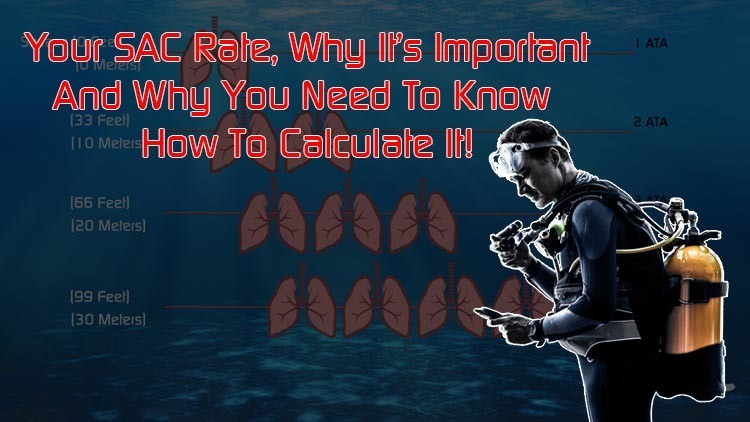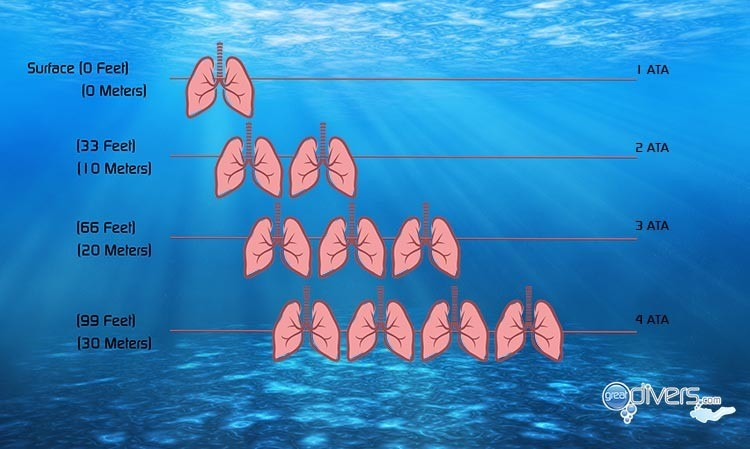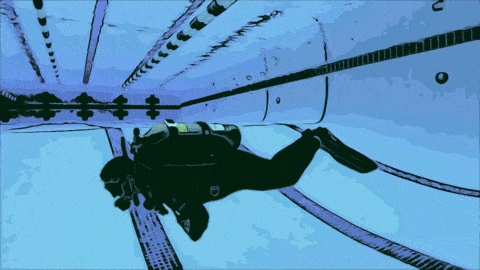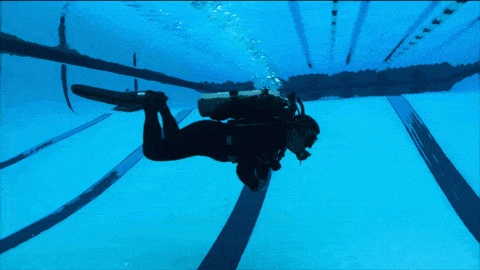# Your SAC Rate, Why It’s Important, and Why You Need To Know How To Calculate It!

February 24, 2021One of the main things that separate experienced and efficient divers from novices is the amount of gas they consume during a dive. Efficient divers will use less gas than someone new and still learning to SCUBA dive. It’s not uncommon as a beginning diver to come back to the boat with just your reserve pressure while your instructor still has their tank half full.  So, how can you measure the amount of gas being consumed by a diver? This is where your SAC rate comes in.

## What is SAC Rate?

Surface Air Consumption (SAC) rate is a measurement used to express how much gas a diver uses while diving. In the imperial system, it is measured in pounds per square inch (PSI) per minute. In the metric system, we measure surface air consumption in BAR per minute. It’s extremely important to understand that the measurement is expressed at the surface. Because atmospheric pressure increases as we dive deeper, the deeper we go, the more gas we consume.

This means that a diver who, for example, breathes 30 PSI per minute on the surface will use 60 PSI at 33 feet (10 meters) and 90 PSI at 66 feet (20 meters). As you can see from the image below, the deeper you go the more gas you will consume.## SAC Rate Calculator

To figure out your SAC rate you’ll need your average depth for a dive, the time you dived for, the amount of gas you observed was used on your submersible pressure gauge (SPG) in PSI or BAR, and the tank you are using during the dive.

Below is a SAC rate calculator. To understand how the math behind how the SAC rate is calculated, the best practices for getting these numbers, and how you can use your SAC rate read on.

• Imperial

• Metric

## Why is SAC Rate Important?

Knowing your SAC rate is important for several reasons, you can use it to:

• Estimate how much gas will be needed for a given dive
• Choose the correct sized tank for a dive
• Track your progress as a diver to see how you improve from dive to dive
• In Technical diving use it to calculate the amount of gas needed for decompression and in case of emergencies

## Dive Computers and your SAC Rate

You can calculate your SAC rate manually, by using a calculator like the one on this page, or you can also get your SAC rate from many dive computers. If your dive computer is air integrated there is a good chance that your dive computer calculates your SAC rate for you.

Even if your dive computer isn’t air integrated, there’s a chance the app or software that connects to your computer can calculate your SAC rate for you.

Because information about your dives is stored by the dive computer, all you need to do is enter the information about the cylinder you used for the dive, your starting pressure, and your ending pressure to have the software calculate your SAC rate.

An important distinction is that not all dive computers / Instructors / textbooks use the term SAC rate. Some call your breathing rate your Respiratory Minute Volume (RMV) or even Surface Consumption Rate (SCR). All these terms are used universally by divers to measure and keep track of your breathing rate on the surface.

## What is RMV in SCUBA Diving?

Respiratory Minute Volume (RMV) is the amount of gas that a diver consumes during a dive expressed as a volume. The measurement is expressed as on the surface. In the imperial system, we use Cubic Feet per Minute to express our RMV. In The metric system, we use Liters Per Minute.

## RMV VS. SAC What’s the difference?

In the diving community the terms Surface Air Consumption (SAC) rate and Respiratory Minute Volume (RMV) rate, are often used interchangeably. Even though the difference is that SAC is expressed as PSI per minute (imperial) or BAR per minute (Metric). While RMV is expressed as a volume (cubic feet per minute or liters per minute).

The problem with using the SAC rate in diving is that the number is dependent on the cylinder being used. So, if for example, you go from using an Aluminum 80 on one dive to a low-pressure steel 95 on a different dive, the SAC rate you calculated using the aluminum 80 won’t be accurate now that you switched tanks. When you standardize the number to RMV the number stays the same no matter what tank you dive with.

I personally teach how to calculate RMV in my SCUBA classes and bypass the entire discussion of SAC rate because it’s a universal number that doesn’t change based on the tank you are using.

Because SAC and RMV are used interchangeably by many, you may find SAC calculators both online, inside of apps, and by dive computers that express the breathing rate as a volume (cubic feet per minute or liters per minute). There is much discussion on internet forums about this topic. The important thing is to understand is what the numbers mean and if you are diving with a buddy and you are comparing numbers to make sure you both are using the same unit of measurement. The semantics about whether SAC should be expressed as pressure or as a volume is really irrelevant when you understand the math and how to use it.

## RMV Calculator

Using the RMV calculator below you can see how you get a value that represents volume instead of PSI or BAR. This value is more useful since the volume of gas that you breathe remains constant with different tanks.

• Imperial

• Metric

## SAC Rate to RMV Calculator

If you already know your SAC rate and the tank you used when you calculated it. You can use the SAC rate to RMV calculator below to convert your SAC rate to RMV. This calculator takes your PSI per minute and converts it to Cubic feet per minute in the imperial system. In the metric calculator it converts BAR per minute to Liters per minute.

• Imperial

• Metric

## Best Practices for Measuring Your SAC or RMV

Regardless of whether you use SAC or RMV to measure your breathing rate, the process of measuring it is the same. You’ll want to pick a dive where you won’t be exerting yourself excessively because of currents or any other conditions. You’ll also want to pick a dive where your depth will be relatively constant. Once you’ve descended and ready to begin measuring, you’ll take a look at your submersible pressure gauge (SPG) and write down your pressure in PSI or BAR. You’ll also want to make a note of the time. Then simply go about your dive as usual. You’ll want to measure for at least 10 minutes (the longer the better). After 10 or more minutes have elapsed make a note of how much gas is left as measured by your SPG and also how long you were diving for. You’ll also need to make a note of average depth during the test. With these numbers handy you can now calculate your SAC or RMV either manually or using the online calculator.

Once you know what your SAC / RMV is, you can use it to estimate the amount of gas you’ll need at depth. Remember that in saltwater you’ll encounter an additional atmosphere of pressure every 33 feet (10 meters). Using the calculator below you can estimate how much gas you’ll use for a given dive. It's important to understand that the calculator below only calculates how much gas you will consume. You also need to factor in your reserve gas in case of an emergency!

• Imperial

• Metric

## What Affects your SAC Rate / RMV Rate?

Many factors influence the rate at which you consume gas from your SCUBA tank. Some of those factors include:

• Your physical size: The larger you are the larger your lungs are and the more gas you will consume as you dive.
• Your exertion level throughout the dive: Swimming fast or against a current are examples of things that will cause you to use more gas.
• Your buoyancy and trim throughout the dive: If your buoyancy is off or you’re not properly trimmed out in the water, you will likely exert yourself more and consume more gas throughout the dive.
• Water Temperature: If you do not have proper thermal protection, your body may begin to shiver and you’ll consume more gas as a consequence of your body trying to stay warm.
• Mental State / Anxiety: If you are anxious, nervous, or not confident in your diving ability this increased anxiousness will often cause you to breathe faster
• Your level of physical fitness: The more cardiovascularly fit you are the more efficient your breathing will be. If you become winded from climbing a flight of stairs, you’ll also likely consume more gas while diving than someone who regularly runs marathons.

## What Is A Good SAC Rate?

A lot of factors go into determining your SAC rate. The single biggest factor is probably your body size. Below is a chart with average SAC / RMV rates for an experienced diver using an Aluminum 80 (Aluminum 11.2 liter) tank. This is for a dive where the diver is not exerting themselves, in tropical waters using a single tank. It is only a guide to get a rough idea of where your SAC / RMV could be. You may be higher or lower than what’s listed on the table. The important thing is to do the measurement and know your measurement. Once you have a starting point you can work on improving your SAC rate.

• Imperial

• Metric

RMV / SAC Rates by Diver in Pounds

﻿Under 140140-180180-220220 Plus
0.4 Cu. Ft. per minute0.5 Cu. Ft. per minute0.6 Cu. Ft. per minute0.7 Cu. Ft. per minute
16 PSI per minute20 PSI per minute24 PSI per minute28 PSI per minute

## How Can I Decrease My SAC Rate?

A good SAC rate is part of being a great diver! There are many things you can do to improve your SAC rate. Many of these suggestions are topics on to themselves and we even have an entire course, much of which is devoted to helping you achieve a lower SAC rate. Below are the top 4 things you can do to decrease your SAC rate.

If you are struggling to stay down because you don’t have enough ballast or have too much ballast and are kicking just to stay off the bottom, then you are surely wasting energy and driving your SAC rate up. Doing a proper buoyancy check, learning where your dump valves are, and mastering buoyancy are the first steps toward a lower SAC rate.

### Learn how to trim your body out properly.

If you are not trimmed out properly in the water, you’ll consume more energy throughout the dive. Notice in the illustration below how the diver to the left encounters more resistance as she tries to kick. This is because she isn’t trimmed out efficiently.## Use the frog kick or modified frog kick more

The frog kick and the modified frog kick require less energy than the flutter kick when you are diving. Because these kicks have a resting phase built-in you end up kicking less to cover the same distance underwater. Mastering the frog kick and the modified frog kick are one of the things you can do to help to lower your breathing rate.### Become More Comfortable Underwater

Your mental state plays a larger role in your breathing rate than most people realize. If you are not completely comfortable underwater it’s likely that your heightened state of anxiety will also raise your breathing rate. Being comfortable with all the basics of SCUBA diving including mask removal and replacement and buddy breathing, in case of emergency, are just the beginning. As you gain experience and become completely comfortable with being underwater your breathing rate will become lower.

## How To Calculate SAC Rate (Formula)

You might be wondering, how are the numbers given by the calculators computed? Below is the explanation so you can understand the math and how the SAC rate works.

### Imperial System SAC Rate Calculation

Computing your SAC rate is fairly straight forward. Remember to follow the guidelines in this article best practices for calculating your SAC / RMV. You can calculate your SAC rate in 3 easy steps. To do so, you’ll need the following:

• Average depth during the test
• Starting and ending PSI during the test
• The amount of time you conducted your SAC rate test for

Let’s assume the following variables for our example:

• Depth = 55 Feet (Saltwater)
• Starting PSI = 2700, Ending PSI = 1800
• Time of Test = 15 minutes

Step 1: Figure out your depth in atmospheres absolute. In this example we assume a dive in saltwater, so we use 33 feet as an atmosphere. If you are diving in freshwater, you’d use 34 feet to calculate an atmosphere. To figure out your depth in atmospheres absolute (ATA) divide your depth by 33 and add 1 to it. So, if for example, you dived to 55 feet, you’ll divide 55 by 33 and add 1. So (55/33) + 1 = 2.68. 2.68 = Your depth in atmospheres absolute (ATA)

Step 2: Figure out how much gas you used in PSI. Assuming you started your test with 2700 PSI and you ended up with 1800 PSI, it means you consumed 900 PSI during your test. 2700 PSI-1800 PSI= 900 PSI.

Step 3: Divide the total PSI used by your ATA and then by the total number of minutes you did the test for. So (900/2.68 = 335.82) and (335.82/15 = 22.38). This gives you your SAC rate. So your SAC rate for this example is 22.38 PSI per minute.

The Formula for calculating your SAC rate is.

((Starting PSI – Ending PSI) / Depth in ATA) / Duration of test) = SAC Rate

*Remember that your SAC rate is dependent on the cylinder you used. Your SAC rate will be different if you use a different cylinder.

### Metric System SAC Rate Calculation

Computing your SAC rate is fairly straight forward in the metric system. Remember to follow the guidelines in this article best practices for calculating your SAC / RMV. You can calculate your SAC rate in 3 easy steps. To do so, you’ll need the following:

• Average depth during the test
• Starting and ending BAR during the test
• The amount of time you conducted your SAC rate test for

Let’s assume the following variables for our example:

• Depth = 17 Meters (Saltwater)
• Starting pressure in BAR = 190, Ending pressure in BAR = 120
• Time of Test = 15 minutes

Step 1: Figure out your depth in atmospheres absolute. To figure out your depth in atmospheres absolute (ATA) divide your depth by 17 Meters By 10 and add 1 to it. So, if for example, you dived to 17 meters, you’ll divide 17 by 10 and add 1. So (17/10) + 1 = 2.7. 2.7 = Your depth in atmospheres absolute (ATA)

Step 2: Figure out how much gas you used in PSI. Assuming you started your test with 190 BAR and you ended up with 120 BAR, it means you consumed 70 BAR during your test. 190 BAR -120 BAR = 70 BAR.

Step 3: Divide the total pressure in BAR you used by your ATA and then by the total number of minutes you did the test for. So (70/2.7 = 25.92) and (25.92/15 = 1.72). This gives you your SAC rate. So, your SAC rate for this example is 1.72 BAR per minute.

The Formula for calculating your SAC rate is.

((Starting BAR – Ending BAR) / Depth in ATA) / Duration of test) = SAC Rate

*Remember that your SAC rate is dependent on the cylinder you used. Your SAC rate will be different if you use a different cylinder.

Do you know your SAC Rate? How do you plan to calculate and use this number? Let us know in the comments below.

Related Posts